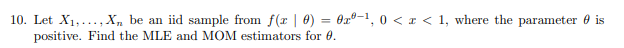# 10. Let X1,..., X„ be an iid sample from f(x | 0) = 0x°-1,positive. Find the MLE and MOM estimators for 0.0 < I < 1, where the parameter 0 is

Question
26 views

Let X1, . . . , Xn be an iid sample from f(x | θ) = θxθ−1  , 0 < x < 1,

where the parameter θ is positive.

Find the MLE and MOM estimators for θ.help_outlineImage Transcriptionclose10. Let X1,..., X„ be an iid sample from f(x | 0) = 0x°-1, positive. Find the MLE and MOM estimators for 0. 0 < I < 1, where the parameter 0 is fullscreen
check_circle

Step 1

Given p.d...

### Want to see the full answer?

See Solution

#### Want to see this answer and more?

Solutions are written by subject experts who are available 24/7. Questions are typically answered within 1 hour.*

See Solution
*Response times may vary by subject and question.
Tagged in
MathStatistics

### Other